# Weighted space

(diff) ← Older revision | Latest revision (diff) | Newer revision → (diff)

function space with weighted norm, weighted class, space with a weight, space of functions with weighted norm

A space of functions with a finite norm (or semi-norm) involving a functional multiplier — the weight. The norm (or semi-norm) of the function is then called the weighted norm (semi-norm), while the weight is also called the weight function of the norm (semi-norm). The introduction of a weight makes it possible to enlarge or restrict ordinary non-weighted normed and semi-normed function spaces consisting of functions with infinite ordinary non-weighted norm (semi-norm). Consider, for example, the weighted space(whereis the weight function), its norm being defined by the formula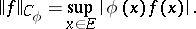If the functionis suitably chosen, the space may be larger or smaller than the space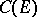with the ordinary norm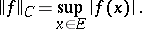For instance, the spacewith the normcontains certain unbounded functions, and includes the spaceof functions bounded on the intervalas a proper subspace. Conversely, the space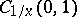with the norm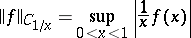is a proper subspace of the space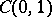. A second example: the semi-normed space with the weighted semi-norm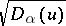, where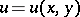and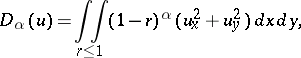, contains, for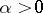, the space of functions with weightless semi-norm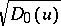as a proper subspace, while forit is a proper subspace of this space.

In most cases the weight function tends to zero or to infinity as its arguments approach a given manifold, which may degenerate to a point, including a point at an infinite distance. Spaces with a weight naturally arise both in the theory of functions when studying ordinary (non-weighted) spaces, and in applications of the theory of functions, e.g. in the theory of boundary value problems for partial differential equations.

The principal problem in the study of weighted spaces is to obtain imbedding theorems for such spaces. In imbedding theorems of the first type the norm of a function is estimated by its norm in another weighted space, assuming both norms are defined for functions with the same domain of definition. Theorems of this type include theorems on the equivalence of norms in a weighted space, in particular concerning norms defined by means of the Fourier–Bessel transform. These include the estimates, in appropriate spaces, of the weighted norms of lower derivatives, in particular of the function itself, in terms of (weighted) norms of higher derivatives for functions vanishing on the boundary of the domain of definition. For instance, these theorems can be used to determine with what weight a function in a given domain is summable if all its higher derivatives belong to a given weighted space. In imbedding theorems of the second type, estimates of the norms of traces of functions on manifolds of smaller dimension in terms of their weighted norms are given.

An important class of weighted spaces consists of function spaces in which the absolute values of all derivatives of the functions, up to an arbitrary order, are summable to a given degree with a power weight. For such cases the imbeddings of weighted spaces have been studied in most detail. For instance, let the weighted space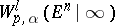consist of the functionson-dimensional Euclidean spacehaving generalized derivatives,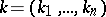, up to order(inclusive) and such that the quantity (which is the norm):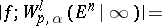remains finite. Here,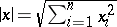,is the-dimensional unit sphere in,,is a real number, and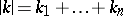. The following imbedding theorem then applies: If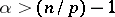,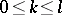, then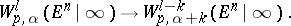Ifis small (i.e. in the case of so-called small degeneration), the weighted spaceshave properties resembling those of weightless spaces: If, then for anythe imbedding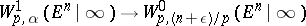holds. If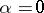, it follows that, for example, the Dirichlet integral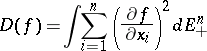(where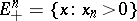is the half-space) is not bounded from below as a functional on the space of functions, belonging to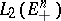, which vanish on the hyperplane, but it is bounded from below on the corresponding weighted space.

If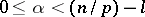, then to each function in the weighted spacethere corresponds a polynomial of degree at leastsuch that the difference between it and the function itself tends to zero as the point tends to infinity along radii.

The trace imbedding theorems for weighted spaces are a generalization of direct and inverse imbedding theorems for ordinary function spaces, and have been studied in depth for weights behaving like some power of the distance from a point in the domain to the boundary of the domain.

Imbedding theorems for weighted spaces are used, first of all, in the theory of degenerate elliptic equations; for them it is possible to give an exact formulation of boundary value problems and to obtain (in dependence on the degree of degeneracy and on the parts of the boundary on which no restrictions are imposed) necessary and sufficient conditions (in terms of properties of boundary values) for the solvability of several boundary value problems; they play an important role in proving the existence and uniqueness of solutions of boundary value problems in their respective weighted spaces and in establishing the stability of this solution in the sense of the energy integral as the boundary values are varied. If the region is unbounded, weighted spaces are also employed in the theory of uniformly elliptic equations.

Imbedding theorems for weighted spaces have found immediate application in solving problems of best extension of a function (or of a system of functions) from a manifold onto the entire space so that the extended function is infinitely differentiable on the complement of the manifold. Here, best extension is understood in the sense of minimal order of growth of derivatives as the point approaches the given manifold. The global degree of smoothness of the function on the manifold gives, for a sufficiently smooth manifold, the largest possible global degree of smoothness of the function which is obtained as a result of extension; for this reason, from some definite order onwards, the derivatives of the extended function will have a finite norm only with a certain weight, i.e. will belong to the respective weighted space.

How to Cite This Entry:
Weighted space. Encyclopedia of Mathematics. URL: http://encyclopediaofmath.org/index.php?title=Weighted_space&oldid=15433
This article was adapted from an original article by L.D. Kudryavtsev (originator), which appeared in Encyclopedia of Mathematics - ISBN 1402006098. See original article